# Strong convergence results for arrays of rowwise pairwise NQD random variables

## Abstract

Let $\left\{{X}_{ni},1\le i\le n,n\ge 1\right\}$ be an array of rowwise pairwise NQD random variables. Some sufficient conditions of complete convergence for weighted sums of arrays of rowwise pairwise NQD random variables are presented without assumption of identical distribution. Our results partially extend the corresponding ones for independent random variables and negatively associated random variables.

MSC:60F15.

## 1 Introduction

Throughout the paper, let $I\left(A\right)$ be the indicator function of the set A. C denotes a positive constant which may be different in various places and ${a}_{n}=O\left({b}_{n}\right)$ stands for ${a}_{n}\le C{b}_{n}$. Denote $logx=lnmax\left(x,e\right)$, where lnx is the natural logarithm.

The concept of complete convergence was introduced by Hsu and Robbins  as follows. A sequence of random variables $\left\{{U}_{n},n\ge 1\right\}$ is said to converge completely to a constant C if ${\sum }_{n=1}^{\mathrm{\infty }}P\left(|{U}_{n}-C|>\epsilon \right)<\mathrm{\infty }$ for all $\epsilon >0$. In view of the Borel-Cantelli lemma, this implies that ${U}_{n}\to C$ almost surely (a.s.). The converse is true if the $\left\{{U}_{n},n\ge 1\right\}$ are independent. Hsu and Robbins  proved that the sequence of arithmetic means of independent and identically distributed (i.i.d.) random variables converges completely to the expected value if the variance of the summands is finite. Since then many authors, such as Spitzer , Baum and Katz , Gut  and so forth, have studied the complete convergence for partial sums and weighted sums of random variables. The main purpose of the present investigation is to provide the complete convergence results for weighted sums of arrays of rowwise pairwise negatively quadrant dependent random variables.

Firstly, let us recall the definition of pairwise negatively quadrant dependent random variables.

Definition 1.1 Two random variables X and Y are said to be negatively quadrant dependent (NQD in short) if for any $x,y\in \mathbb{R}$,

$P\left(X\le x,Y\le y\right)\le P\left(X\le x\right)P\left(Y\le y\right).$
(1.1)

A sequence of random variables $\left\{{X}_{n},n\ge 1\right\}$ is said to be pairwise NQD if ${X}_{i}$ and ${X}_{j}$ are NQD for all $i,j\in \mathbb{N}$ and $i\ne j$.

An array of random variables $\left\{{X}_{ni},1\le i\le n,n\ge 1\right\}$ is called rowwise pairwise NQD random variables if for every $n\ge 1$, $\left\{{X}_{ni},1\le i\le n\right\}$ are pairwise NQD random variables.

The concept of pairwise NQD was introduced by Lehmann . Obviously, a sequence of pairwise NQD random variables is a family of very wide scope, which contains a pairwise independent random variable sequence and a negatively orthant dependent (NOD) random variable sequence as special cases. For more details about NOD random variables, one can refer to Joag-Dev and Proschan , Wang et al. [7, 8] and so forth. Many known types of negative dependence such as negative upper (lower) orthant dependence and negative association (see Joag-Dev and Proschan ) have been developed on the basis of this notion. Among them, the negatively associated class is the most important and special case of a pairwise NQD sequence. So, it is very significant to study probabilistic properties of this wider pairwise NQD class. Since Lehmann’s article appeared, a number of limit theorems for pairwise NQD random variables have been established. For example, Matula  obtained the Kolmogorov strong law of large numbers for a pairwise NQD random variable sequence with identical distribution, Wang et al.  and Wu  investigated some limit properties for such a sequence, Li and Wang  obtained the central limit theorem, Gan and Chen  studied further some limit properties for the pairwise NQD sequence without limitation of an identically distributed condition and obtained Baum-Katz (Baum and Katz ) type complete convergence and the strong stability of Jamison (Jamison et al. ) type weighted sums for the pairwise NQD sequence which may have different distributions, Huang et al.  studied the complete convergence for sequences of pairwise NQD random variables and so forth.

Our goal in this paper is to further study the complete convergence for weighted sums of arrays of rowwise pairwise NQD random variables under some moment conditions. We will give some sufficient conditions for complete convergence for an array of rowwise pairwise NQD random variables without assumption of identical distribution. The results presented in this paper are obtained by using the truncated method and the generalized Kolmogorov type inequality of pairwise NQD random variables.

Definition 1.2 An array of random variables $\left\{{X}_{ni},i\ge 1,n\ge 1\right\}$ is said to be stochastically dominated by a random variable X if there exists a positive constant C such that

$P\left(|{X}_{ni}|>x\right)\le CP\left(|X|>x\right)$
(1.2)

for all $x\ge 0$, $i\ge 1$ and $n\ge 1$.

The following lemmas are useful for the proof of the main results.

Lemma 1.1 (cf. Lehmann )

Let X and Y be NQD, then

1. (i)

$EXY\le EXEY$;

2. (ii)

$P\left(X>x,Y>y\right)\le P\left(X>x\right)P\left(Y>y\right)$, for any $x,y\in R$;

3. (iii)

If f and g are both nondecreasing (or nonincreasing) functions, then $f\left(X\right)$ and $g\left(Y\right)$ are NQD.

Lemma 1.2 (cf. Wu )

Let $\left\{{X}_{n},n\ge 1\right\}$ be a sequence of pairwise NQD random variables with $E{X}_{n}=0$ and $E{X}_{n}^{2}<\mathrm{\infty }$ for every $n\ge 1$. Denote ${T}_{j}\left(k\right)={\sum }_{i=j+1}^{j+k}{X}_{i}$, $j\ge 0$, $k\ge 1$. Then, for every $n\ge 1$,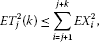(1.3)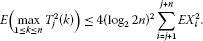(1.4)

Lemma 1.3 Let $\left\{{X}_{n},n\ge 1\right\}$ be a sequence of random variables which is stochastically dominated by a random variable X. For any $\alpha >0$ and $b>0$, the following two statements hold:(1.5)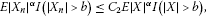(1.6)

where ${C}_{1}$ and ${C}_{2}$ are positive constants.

Proof The proof can be found in Wu . So, we omit the details. □

The following two lemmas are from Sung .

Lemma 1.4 Let X be a random variable and $\left\{{a}_{ni},1\le i\le n,n\ge 1\right\}$ be an array of constants satisfying

$\sum _{i=1}^{n}|{a}_{ni}{|}^{\alpha }=O\left(n\right)$
(1.7)

for some $\alpha >0$. Let ${b}_{n}={n}^{1/\alpha }{\left(logn\right)}^{1/\gamma }$ for some $\gamma >0$. Then

$\sum _{n=1}^{\mathrm{\infty }}{n}^{-1}\sum _{i=1}^{n}P\left(|{a}_{ni}X|>{b}_{n}\right)\le \left\{\begin{array}{cc}CE{|X|}^{\alpha },\hfill & \mathit{\text{for}}\phantom{\rule{0.5em}{0ex}}\alpha >\gamma ,\hfill \\ CE{|X|}^{\alpha }log\left(1+|X|\right),\hfill & \mathit{\text{for}}\phantom{\rule{0.5em}{0ex}}\alpha =\gamma ,\hfill \\ CE{|X|}^{\gamma },\hfill & \mathit{\text{for}}\phantom{\rule{0.5em}{0ex}}\alpha <\gamma .\hfill \end{array}$

Lemma 1.5 Let X be a random variable and $\left\{{a}_{ni},1\le i\le n,n\ge 1\right\}$ be an array of constants satisfying ${a}_{ni}=0$ or ${a}_{ni}>1$, and ${\sum }_{i=1}^{n}|{a}_{ni}{|}^{\alpha }\le n$ some $\alpha >0$. Let ${b}_{n}={n}^{1/\alpha }{\left(logn\right)}^{1/\alpha }$. If $q>\alpha$, then

$\sum _{n=1}^{\mathrm{\infty }}{n}^{-1}{b}_{n}^{-q}\sum _{i=1}^{n}E|{a}_{ni}X{|}^{q}I\left(|{a}_{ni}X|\le {b}_{n}\right)\le CE{|X|}^{\alpha }log\left(1+|X|\right).$

## 2 Main results

Theorem 2.1 Let $\left\{{X}_{ni},1\le i\le n,n\ge 1\right\}$ be an array of rowwise pairwise NQD random variables which is stochastically dominated by a random variable X and $\left\{{a}_{ni},1\le i\le n,n\ge 1\right\}$ be an array of constants satisfying (1.7) for some $0<\alpha <2$. Let ${b}_{n}\doteq {n}^{1/\alpha }{\left(logn\right)}^{1/\alpha }$. If $E{X}_{ni}=0$ for $1<\alpha <2$ and $E{|X|}^{\alpha }log\left(1+|X|\right)<\mathrm{\infty }$, then for any $\epsilon >0$,

$\sum _{n=1}^{\mathrm{\infty }}{n}^{-1}P\left(|\sum _{i=1}^{n}{a}_{ni}{X}_{ni}|>\epsilon {b}_{n}\right)<\mathrm{\infty }.$
(2.1)

Proof Without loss of generality, we may assume that ${\sum }_{i=1}^{n}|{a}_{ni}{|}^{\alpha }\le n$ and ${a}_{ni}\ge 0$ for all $1\le i\le n$ and $n\ge 1$. For fixed $n\ge 1$, define for $1\le i\le n$ that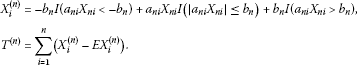It is easy to check that for any $\epsilon >0$,

$\left(|\sum _{i=1}^{n}{a}_{ni}{X}_{ni}|>\epsilon {b}_{n}\right)\subset \left(\underset{1\le i\le n}{max}|{a}_{ni}{X}_{ni}|>{b}_{n}\right)\cup \left(|\sum _{i=1}^{n}{X}_{i}^{\left(n\right)}|>\epsilon {b}_{n}\right),$

which implies that

$\begin{array}{rcl}P\left(|\sum _{i=1}^{n}{a}_{ni}{X}_{ni}|>\epsilon {b}_{n}\right)& \le & P\left(\underset{1\le i\le n}{max}|{a}_{ni}{X}_{ni}|>{b}_{n}\right)+P\left(|\sum _{i=1}^{n}{X}_{i}^{\left(n\right)}|>\epsilon {b}_{n}\right)\\ \le & \sum _{i=1}^{n}P\left(|{a}_{ni}{X}_{ni}|>{b}_{n}\right)+P\left(|{T}^{\left(n\right)}|>\epsilon {b}_{n}-|\sum _{i=1}^{n}E{X}_{i}^{\left(n\right)}|\right)\\ \le & C\sum _{i=1}^{n}P\left(|{a}_{ni}X|>{b}_{n}\right)+P\left(|{T}^{\left(n\right)}|>\epsilon {b}_{n}-|\sum _{i=1}^{n}E{X}_{i}^{\left(n\right)}|\right).\end{array}$

Firstly, we will show that

(2.2)

When $1<\alpha <2$, we have by $E{X}_{ni}=0$, (1.6) of Lemma 1.3 and Markov’s inequality that

(2.3)

When $0<\alpha \le 1$, we have by (1.5) of Lemma 1.3 and Markov’s inequality that

(2.4)

By (2.3) and (2.4), we can get (2.2) immediately. Hence, for n large enough,

$P\left(|\sum _{i=1}^{n}{a}_{ni}{X}_{ni}|>\epsilon {b}_{n}\right)\le C\sum _{i=1}^{n}P\left(|{a}_{ni}X|>{b}_{n}\right)+P\left(|{T}^{\left(n\right)}|>\frac{\epsilon }{2}{b}_{n}\right).$

To prove (2.1), we only need to show that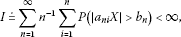(2.5)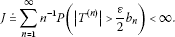(2.6)

By Lemma 1.4 and the condition $E{|X|}^{\alpha }log\left(1+|X|\right)<\mathrm{\infty }$, we can see that

$I\le CE{|X|}^{\alpha }log\left(1+|X|\right)<\mathrm{\infty },$

which implies (2.5).

For fixed $n\ge 1$, it is easily seen that $\left\{{X}_{i}^{\left(n\right)}-E{X}_{i}^{\left(n\right)},1\le i\le n\right\}$ are still pairwise NQD with mean zero by (iii) of Lemma 1.1. Hence, it follows from Markov’s inequality, (1.3) of Lemma 1.2 and (1.5) of Lemma 1.3 that

$\begin{array}{rcl}J& \le & C\sum _{n=1}^{\mathrm{\infty }}{n}^{-1}{b}_{n}^{-2}E|{T}^{\left(n\right)}{|}^{2}\le C\sum _{n=1}^{\mathrm{\infty }}{n}^{-1}{b}_{n}^{-2}\sum _{i=1}^{n}E|{X}_{i}^{\left(n\right)}{|}^{2}\\ \le & C\sum _{n=1}^{\mathrm{\infty }}{n}^{-1}{b}_{n}^{-2}\sum _{i=1}^{n}\left[E{|{a}_{ni}{X}_{ni}|}^{2}I\left(|{a}_{ni}{X}_{ni}|\le {b}_{n}\right)+{b}_{n}^{2}P\left(|{a}_{ni}{X}_{ni}|>{b}_{n}\right)\right]\\ \le & C\sum _{n=1}^{\mathrm{\infty }}{n}^{-1}{b}_{n}^{-2}\sum _{i=1}^{n}\left[E{|{a}_{ni}X|}^{2}I\left(|{a}_{ni}X|\le {b}_{n}\right)+{b}_{n}^{2}P\left(|{a}_{ni}X|>{b}_{n}\right)\right]\\ =& C\sum _{n=1}^{\mathrm{\infty }}{n}^{-1}{b}_{n}^{-2}\sum _{i=1}^{n}E{|{a}_{ni}X|}^{2}I\left(|{a}_{ni}X|\le {b}_{n}\right)+C\sum _{n=1}^{\mathrm{\infty }}{n}^{-1}\sum _{i=1}^{n}P\left(|{a}_{ni}X|>{b}_{n}\right)\\ \doteq & {J}_{1}+{J}_{2}.\end{array}$
(2.7)

By Lemma 1.4 and the condition $E{|X|}^{\alpha }log\left(1+|X|\right)<\mathrm{\infty }$ again, we can see that

${J}_{2}\le CE{|X|}^{\alpha }log\left(1+|X|\right)<\mathrm{\infty }.$

To prove ${J}_{1}<\mathrm{\infty }$, we divide $\left\{{a}_{ni},1\le i\le n\right\}$ into three subsets $\left\{{a}_{ni}:|{a}_{ni}|\le 1/{\left(logn\right)}^{s}\right\}$, $\left\{{a}_{ni}:1/{\left(logn\right)}^{s}<|{a}_{ni}|\le 1\right\}$, $\left\{{a}_{ni}:|{a}_{ni}|>1\right\}$, where $s=\frac{1}{2-\alpha }$. Hence

$\begin{array}{rcl}{J}_{1}& =& C\sum _{n=1}^{\mathrm{\infty }}{n}^{-1}{b}_{n}^{-2}\sum _{i:|{a}_{ni}|\le 1/{\left(logn\right)}^{s}}E{|{a}_{ni}X|}^{2}I\left(|{a}_{ni}X|\le {b}_{n}\right)\\ +C\sum _{n=1}^{\mathrm{\infty }}{n}^{-1}{b}_{n}^{-2}\sum _{i:1/{\left(logn\right)}^{s}<|{a}_{ni}|\le 1}E{|{a}_{ni}X|}^{2}I\left(|{a}_{ni}X|\le {b}_{n}\right)\\ +C\sum _{n=1}^{\mathrm{\infty }}{n}^{-1}{b}_{n}^{-2}\sum _{i:|{a}_{ni}|>1}E{|{a}_{ni}X|}^{2}I\left(|{a}_{ni}X|\le {b}_{n}\right)\\ \doteq & {J}_{11}+{J}_{12}+{J}_{13}.\end{array}$

Note that

$\sum _{i:|{a}_{ni}|\le 1/{\left(logn\right)}^{s}}{|{a}_{ni}|}^{\alpha }\le n{\left(logn\right)}^{-s\alpha },$

we have by $E{|X|}^{\alpha }<\mathrm{\infty }$ that

Note that $s=\frac{1}{2-\alpha }$ and

$\sum _{i:1/{\left(logn\right)}^{s}<|{a}_{ni}|\le 1}{|{a}_{ni}|}^{2}\le n,$

we have by $E{|X|}^{\alpha }<\mathrm{\infty }$ again that

By Lemma 1.5 and the condition $E{|X|}^{\alpha }log\left(1+|X|\right)<\mathrm{\infty }$, we can see that

${J}_{13}\doteq C\sum _{n=1}^{\mathrm{\infty }}{n}^{-1}{b}_{n}^{-2}\sum _{i:|{a}_{ni}|>1}E{|{a}_{ni}X|}^{2}I\left(|{a}_{ni}X|\le {b}_{n}\right)\le CE{|X|}^{\alpha }log\left(1+|X|\right)<\mathrm{\infty }.$

Therefore, ${J}_{1}<\mathrm{\infty }$ follows from the statements above. This completes the proof of the theorem. □

Remark 2.1 The key to the proof of Theorem 2.1 is the Kolmogorov type inequality for pairwise NQD random variables. For many sequences of random variables, such as independent sequence, negatively associated sequence (see Shao ), negatively dependent sequence (see Asadian et al. ), ${\rho }^{\ast }$-mixing sequence (see Utev and Peligrad ), φ-mixing sequence (see Wang et al. ) and so forth, the Kolmogorov type inequality also holds. So, Theorem 2.1 also holds for these sequences.

Remark 2.2 Theorem 2.1 only holds for $0<\alpha <2$. It is still an open question whether Theorem 2.1 holds for $\alpha =2$. In addition, it is still an open question whether

$\sum _{n=1}^{\mathrm{\infty }}{n}^{-1}P\left(\underset{1\le j\le n}{max}|\sum _{i=1}^{j}{a}_{ni}{X}_{ni}|>\epsilon {b}_{n}\right)<\mathrm{\infty }$

holds for any $\epsilon >0$ under the conditions of Theorem 2.1. The authors suggest that a solution can be obtained if a better moment inequality than the one presented above in Lemma 1.2 could be established.

## References

1. Hsu PL, Robbins H: Complete convergence and the law of large numbers. Proc. Natl. Acad. Sci. USA 1947, 33(2):25–31. 10.1073/pnas.33.2.25

2. Spitzer FL: A combinatorial lemma and its application to probability theory. Trans. Am. Math. Soc. 1956, 82(2):323–339. 10.1090/S0002-9947-1956-0079851-X

3. Baum LE, Katz M: Convergence rates in the law of large numbers. Trans. Am. Math. Soc. 1965, 120: 108–123. 10.1090/S0002-9947-1965-0198524-1

4. Gut A: Complete convergence for arrays. Period. Math. Hung. 1992, 25(1):51–75. 10.1007/BF02454383

5. Lehmann EL: Some concepts of dependence. Ann. Math. Stat. 1966, 37: 1137–1153. 10.1214/aoms/1177699260

6. Joag-Dev K, Proschan F: Negative association of random variables with applications. Ann. Stat. 1983, 11: 286–295. 10.1214/aos/1176346079

7. Wang XJ, Hu SH, Yang WZ, Ling NX: Exponential inequalities and inverse moment for NOD sequence. Stat. Probab. Lett. 2010, 80(5–6):452–461. 10.1016/j.spl.2009.11.023

8. Wang XJ, Hu SH, Shen AT, Yang WZ: An exponential inequality for a NOD sequence and a strong law of large numbers. Appl. Math. Lett. 2011, 24: 219–223. 10.1016/j.aml.2010.09.007

9. Matula P: A note on the almost sure convergence of sums of negatively dependent random variables. Stat. Probab. Lett. 1992, 15: 209–213. 10.1016/0167-7152(92)90191-7

10. Wang YB, Su C, Liu XG: On some limit properties for pairwise NQD sequences. Acta Math. Appl. Sin. 1998, 21: 404–414.

11. Wu QY: Convergence properties of pairwise NQD random sequences. Acta Math. Sin. 2002, 45: 617–624.

12. Li YX, Wang JF: An application of Stein’s method to limit theorems for pairwise negative quadrant dependent random variables. Metrika 2008, 67(1):1–10.

13. Gan SX, Chen PY: Some limit theorems for sequences of pairwise NQD random variables. Acta Math. Sci. 2008, 28(2):269–281.

14. Jamison B, Orey S, Pruitt W: Convergence of weighted averages of independent random variables. Z. Wahrscheinlichkeitstheor. Verw. Geb. 1965, 4: 40–44. 10.1007/BF00535481

15. Huang HW, Wang DC, Wu QY, Zhang QX: A note on the complete convergence for sequences of pairwise NQD random variables. Arch. Inequal. Appl. 2011., 2011: Article ID 92. doi:10.1186/1029–242X-2011–92

16. Wu QY: Probability Limit Theory for Mixing Sequences. Science Press of China, Beijing; 2006.

17. Sung SH:On the strong convergence for weighted sums of ${\rho }^{\ast }$-mixing random variables. Stat. Pap. 2012. doi:10.1007/s00362–012–0461–2

18. Shao QM: A comparison theorem on moment inequalities between negatively associated and independent random variables. J. Theor. Probab. 2000, 13(2):343–356. 10.1023/A:1007849609234

19. Asadian N, Fakoor V, Bozorgnia A: Rosenthal’s type inequalities for negatively orthant dependent random variables. JIRSS 2006, 5(1–2):69–75.

20. Utev S, Peligrad M: Maximal inequalities and an invariance principle for a class of weakly dependent random variables. J. Theor. Probab. 2003, 16(1):101–115. 10.1023/A:1022278404634

21. Wang XJ, Hu SH, Yang WZ, Shen Y: On complete convergence for weighted sums of φ -mixing random variables. Arch. Inequal. Appl. 2010., 2010: Article ID 372390

## Acknowledgements

The authors are most grateful to the editor Andrei Volodin and the anonymous referee for careful reading of the manuscript and valuable suggestions which helped in improving an earlier version of this paper. This work was supported by the Project of the Feature Specialty of China (TS11496) and the Scientific Research Projects of Fuyang Teachers College (2009FSKJ09).

## Author information

Authors

### Corresponding author

Correspondence to Xiaofeng Tang.

### Competing interests

The author declares that they have no competing interests.

## Rights and permissions

Reprints and Permissions

Tang, X. Strong convergence results for arrays of rowwise pairwise NQD random variables. J Inequal Appl 2013, 102 (2013). https://doi.org/10.1186/1029-242X-2013-102